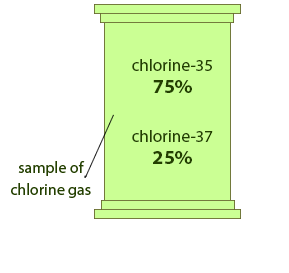## Relative Atomic mass

Different types of atoms have different masses. This mass is too small to measure using a conventional scale, therefore we compare their masses to each other. A carbon atom having a mass number 12, i.e. (12C) is taken as standard for this comparison and its relative atomic mass is 12.

The relative atomic mass of an element compares the mass of atoms of the element with the 12C isotope.

It is written as Ar or R.A.M.

Some of the elements exist in nature as a mixture of their isotopes in specific proportions. The R.A.M of such elements is the average mass of the different proportions of each isotope in the mixture.

### R.A.M. of some elements:

 hydrogen 1 oxygen 16 copper 63.5 iron 55.8

It’s important to note that atomic number and mass number are always whole numbers because they are based on the number of sub-atomic particles, while the R.A.M. can have fractions because it is the average mass of different isotopes.

## ExampleA sample of chlorine gas is a mixture of 2 isotopes, chlorine-35 and chlorine-37. These isotopes occur in specific proportions in the sample i.e. 75% chlorine-35 and 25% chlorine-37. Calculate the R.A.M. of chlorine in the sample.

## Solution

The average mass, or R.A.M. of chlorine can be calculated using the following equation:

 R.A.M. = (mass of isotope-A  x  % of isotope-A) + (mass of isotope-B  x  % of isotope-B) 100

 = (35 x 75) + (37 x 25) 100

 = 3550 100

 R.A.M. = 35.5